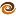# EmptyCrate

#### About Me | Contact Me | Training |ChaiScript.com | YouTube Channel

Neither C++ Coding Standardsnor Effective C++addresses the question of which float point type is best to use and in what situations. There are three floating point types in C and C++:

1. `float`
2. `double`
3. `long double`

What the Standard Has to Say There are exactly two guarantees provided by the standard:

`precision(float) <= precision(double) <= precision(long double)`. We are guaranteed that `float` has no more precision than `double` and `double` has no more precision than `long double`. It is possible for all three to be the same data type in a given implementation.

The default floating point type is `double`, that is, `typeid(3.3)` is `double`.

• f suffix

If you want a `float` constant, you must specify this with the `f` suffix: `3.3f`.

• L suffix

Similarly, a `long double` constant must be specified with the `L` suffix: `3.3L`.

Guidance Provided by Stroustrup

In all of my C++ resources, the only guidance I can find is in the C++ Programming Language by Bjarne Stroustrup (the creator of C++).

“The exact meaning of single-, double-, and extended-precision is implementation-defined. Choosing the right precision for a problem where the choice matters requires significant understanding of floating-point computation. If you don’t have that understanding, get advice, take the time to learn, or use double and hope for the best.” [emphasis added]

Standard Library Implementation

Where there is only one version of a standard library floating point operation, the library defaults to working with `double`. This includes the functions `atof` and `strtod`. In C89 the only data type supported by all math.h functions was `double`. Performance On modern hardware `double` outperforms `float` in every case. In the higher optimization levels `long double` even outperforms float. The test code was compiled with the command line

``````g++ floatdouble.cpp -std=c++0x -O3 -march=native
``````

Type name: f Size in bytes: 4 Summation time in s: 2.82 summed value: 6.71089e+07 // float Type name: d Size in bytes: 8 Summation time in s: 2.78585 summed value: 6.6e+09 // double Type name: e Size in bytes: 16 Summation time in s: 2.76812 summed value: 6.6e+09 // long double

The test code was:

``````#include <chrono>
#include <vector>
#include <iostream>
#include <typeinfo>

template
T sum(int num_times, T value)
{
T val=0;

std::chrono::high_resolution_clock::time_point t1 = std::chrono::high_resolution_clock::now();
for (int i = 0; i < num_times; ++i)
{
val += value;
}
std::chrono::high_resolution_clock::duration d = std::chrono::high_resolution_clock::now() - t1;

std::cout << "Type name: " << typeid(T).name() << " Size in bytes: " << sizeof(T) << " Summation time in s: " << std::chrono::duration_cast>(d).count();

return val;
}

int main()
{
std::cout << " summed value: " << sum(2000000000, 3.3) << std::endl;
std::cout << " summed value: " << sum(2000000000, 3.3) << std::endl;
std::cout << " summed value: " << sum(2000000000, 3.3) << std::endl;
std::cout << " summed value: " << sum(2000000000, 3.3) << std::endl;
std::cout << " summed value: " << sum(2000000000, 3.3) << std::endl;
std::cout << " summed value: " << sum(2000000000, 3.3) << std::endl;
std::cout << " summed value: " << sum(2000000000, 3.3) << std::endl;
}
``````

Conclusion

`double` should be your preferred floating point type in nearly every situation.

1. It’s faster.
2. It’s the default in C and C++.
3. It’s more portable and the default across all C and C++ library functions.
4. It’s significantly higher precision. The answer above is outright wrong (off by 2 orders of magnitude) for float. My simple 3.3 summation example quickly loses precision. In a smaller case, summing the value 3.3 2000 times results in: 6599.89 when using `float` instead of the correct answer of 6600.
5. Stroustrup recommends it.

There is exactly one case where you should use `float` instead of `double`

1. It’s smaller. On 64bit hardware with a modern gcc, `double` is 8 bytes and `float` is 4 bytes.

Similarly, there is exactly one time you would need to use `long double`.

1. It’s very high precision. You might have an application that needs the absolutely most correct floating point precision you can get today.

Finally, and perhaps most importantly: 64bit floating point has been the standard supported in Intel compatible CPU’s supporting the SSE2 instruction set since 2001. On most platforms `double` uses this same 64 SSE2 (IEEE64 bit) type.

My Videos

Related